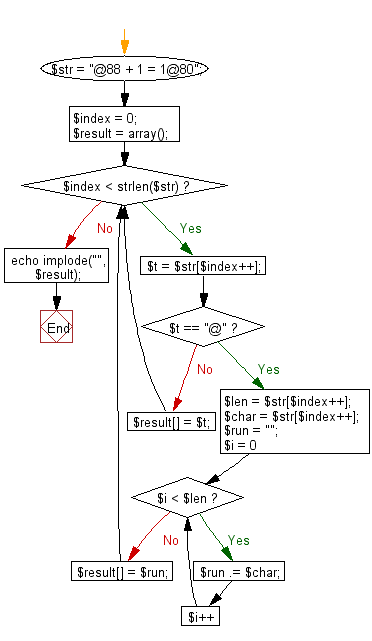﻿ PHP Exercise: Restore the original string by entering the compressed string with this rule - w3resource

# PHP Exercises: Restore the original string by entering the compressed string with this rule

## PHP: Exercise-72 with Solution

When character are consecutive in a string , it is possible to shorten the character string by replacing the character with a certain rule. For example, in the case of the character string YYYYY, if it is expressed as # 5 Y, it is compressed by one character.
Write a PHP program to restore the original string by entering the compressed string with this rule. However, the # character does not appear in the restored character string.
Note: The original sentences are uppercase letters, lowercase letters, numbers, symbols, less than 100 letters, and consecutive letters are not more than 9 letters.

Input: Multiple character strings are given. One string is given per line

Sample Solution: -

PHP Code:

``````<?php
\$str  = "@88 + 1 = [email protected]";
\$index = 0;
\$result = array();
while(\$index < strlen(\$str)) {
\$t = \$str[\$index++];
if (\$t == "@") {
\$len = \$str[\$index++];
\$char = \$str[\$index++];
\$run = "";
for (\$i = 0; \$i < \$len; \$i++) {
\$run .= \$char;
}
\$result[] = \$run;
} else {
\$result[] = \$t;
}
}
echo implode("", \$result);
?>
```
```

Sample Input:
@88 + 1 = [email protected]

Sample Output:

`88888888 + 1 = 100000000`

Flowchart:PHP Code Editor:

Have another way to solve this solution? Contribute your code (and comments) through Disqus.

What is the difficulty level of this exercise?

Test your Programming skills with w3resource's quiz.

﻿

## PHP: Tips of the Day

Mutates the original array to filter out the values specified

Example:

```<?php
function tips_pull(&\$items, ...\$params)
{
\$items = array_values(array_diff(\$items, \$params));
return \$items;
}

\$items = ['x', 'y', 'z', 'x', 'y', 'z'];
print_r(tips_pull(\$items, 'y', 'z'));
?>
```

Output:

```Array
(
 => x
 => x
)
```